Polyrects (rectangles)

Polyrects are defined in WolframMathWorld as polyforms obtained from a rectangular grid. Here we use a rectangle with sides in the ratio 5:4 but any other could be used.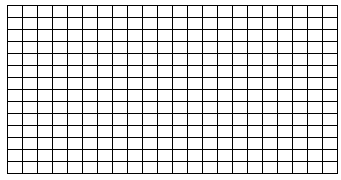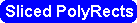The following table gives numbers of polyrects.Note: For every a x b rectangle (or set of rectangles) shown here a solution for the b x a rectangle (or set of rectangles) can be obtained by reducing the horizontal axis by 20%; increasing the y-axis by 25% and rotating through 90o.

Tetrarects

The nine tetrarects cannot form a recangle but with one piece used twice 4x10 and 5x8 rectangles are possible.The 13 one-sided pieces cannot form a rectangle but can form the following figure.Pentarects

The 21 pentarects are shown here in a numbers of constructions.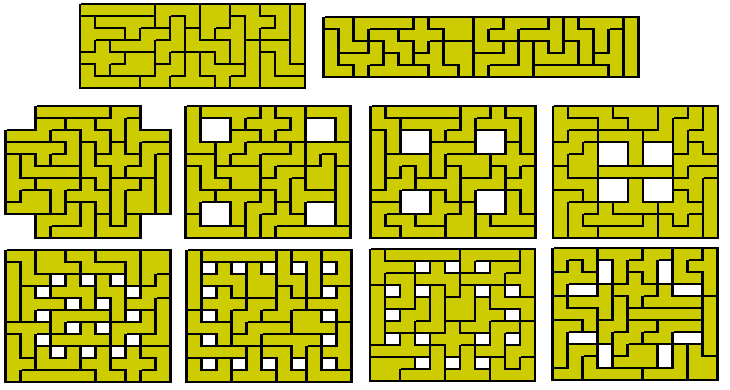Alexandre Owen Muniz has noted that the pentarects can form three 5x7 rectangles and if we use a ratio of 5:7 for the rectangle they appear as three squares. He also has some more information on his Puzzle Zapper Blog page.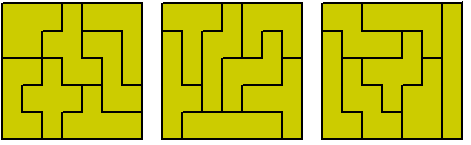The oneisided set consists of 35 pieces shown here in a 7x25 and a 5x35 rectangle.These pieces can also make fivefold replicas of a heptarect. It is likely that all 208 problems are possible.Hexarects

The 68 hexarects cannot make a rectangle. All but two of the pieces have rotational equivalents which are different. These 66 pieces can form three 11x12 rectangles as shown.The remaining two pieces which do not have rotational equivalents other than themselves can be divided into a T-tetromino and a Domino (figure 1 below). In using these two pieces then the T's can be either in parallel or perpendicular orientations.

Using parallel orientations we see that the two T's can combine to form a shape with two vertical and two horizontal dominoes. However the remaing dominoes from the pieces are perpendicular to each other (figure 2).

If the T's are perpendicular then the combination will form 3 dominoes in one direction an one perpendicular while now the remaining two dominoes are paralle (figure 3)l.

Since any rectangle to be formed must be divible into dominoes with even numbers in each direction no rectangle is possible if these two pieces are included.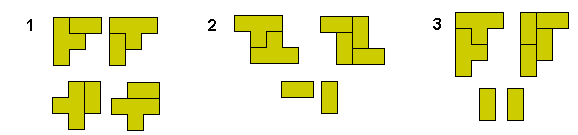This is further emphasised by the constructions below where three 6x23 rectangles are formed when one piece is used twice. Notice that in each case the extra piece is formed from two dominoes in one direction and one perpendicular.There are 48 pieces which are balanced (3-3) in terms of chessboard colouring. These are shown below.The one-sided set consists of 120 pieces. They are shown hers in four 12x15, five 12x12,six 10x12 and eight 9x10 rectanglesSets of similar figures are possible to make. These are based on 120=12+22+32+32+42+62and 120=22+22+22+62+62+62.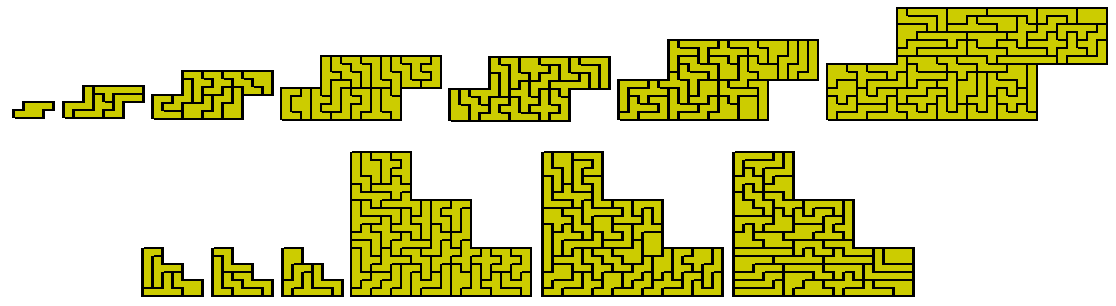Two 12x15 and two 15x12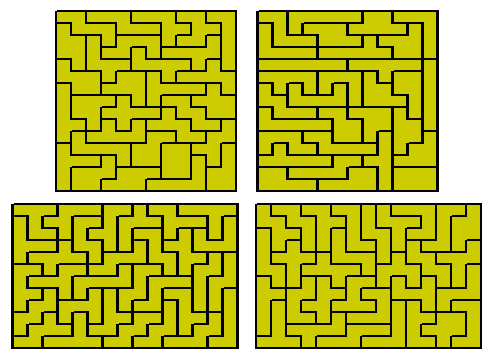Heptarects

This set consists of 208 pieces one of which has an internal hole.Sets of four congruent figures can also be made.The 207 pieces without a hole can form a number of rectangles as shown in the table below. the 23 7x9 rectangles cannot be made owing to the fact that there are insufficient pieces which can form the required 736 edges.The 9x161 rectangle and three 21x23 rectangles are shown here.There are 392 one-sided pieces two of which have and internal hole. They are shown here in eight rectangles with two holes in each.The remaining pices can form a large number of rectangles as shown in the table below. Also shown is the various sets of rectangles which may be formed.Here we have two 13x105 rectangles;.here two 15x91 rectangles;two 21x65 rectangles;and three 10x91 rectangles.The fifteen 13x14 rectangles below will form three 13x70, three 26x35; five13x42 or five 14x39 rectangles .Octorects

Of the 730 two-sided octorects eleven have an internal hole leaving 719 without a hole. As this is prime the only possible rectangle would be an 8x719.